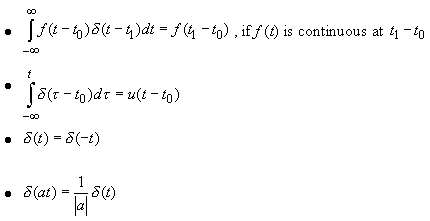# Singularity Functions

### The Unit Step Function

We already defined the unit step function u(t) as: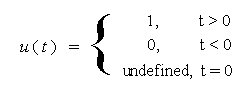Ex: Find and plot u(t - t0) and u(t - t1)

Ex. Define a block function (window) as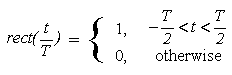Then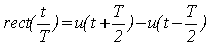Example:    Plot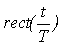### The Unit Impulse Function

 Now let's look at a signal: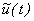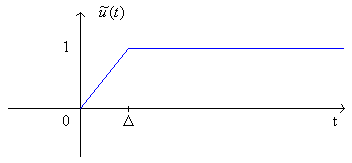What is its derivative? Define it as: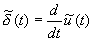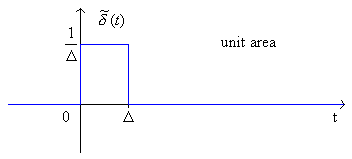which has unit area.

 Now,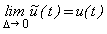So what if we take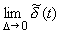?

The pulse height gets higher and higher and its width goes to 0, but its area is still 1!

So define δ(t) as the unit impulse: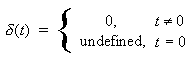AND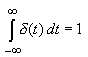or equivalently,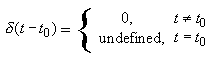AND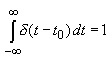Also,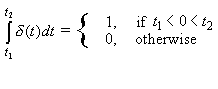δ(t) can be considered to be the derivative of u(t) but only in a restricted sense since u(t) is a discontinuous function.

Note that the impulse function is not a true function since it is not defined for all values of t. It's a "generalized function." But its idealization will allow us to derive many interesting results.

### Unit Impulse Properties

1.    Scaling

(t) is an impulse with weight or area K: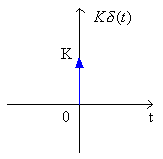2.    Multiplication of a function x(t) (that is continuous at 0) by an impulse δ(t):

We get an impulse with area or weight x(0).

3.    Time Shift of an impulse

y(t) = x(t)δ(t-t0)So we get an impulse with weight equal to the value of x(t) where the impulse is located:

y(t) = x(t0)δ(t-t0)

Example What is Kx(t)δ(t-t0)?

Example What is 3u(t-1)δ(t) ?

***SIFTING PROPERTY***

What if we multiply a function by an impulse and then integrate?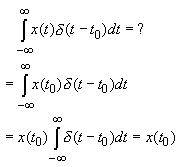We integrate out the time variable so the integral is just equal to a number (or later on, a function). We'll see this many times.In this case, the impulse δ(t-t0) is defined by the integral (as long as the function x(t) is continuous at t0).

Ex.

ALSO: